# Resources tagged with: Patterned numbers

Filter by: Content type:
Age range:
Challenge level:

### There are 32 results

Broad Topics > Numbers and the Number System > Patterned numbers### Lastly - Well

##### Age 11 to 14 Challenge Level:

What are the last two digits of 2^(2^2003)?### Sept 03

##### Age 11 to 14 Challenge Level:

What is the last digit of the number 1 / 5^903 ?### Like Powers

##### Age 11 to 14 Challenge Level:

Investigate $1^n + 19^n + 20^n + 51^n + 57^n + 80^n + 82^n$ and $2^n + 12^n + 31^n + 40^n + 69^n + 71^n + 85^n$ for different values of n.### Power Crazy

##### Age 11 to 14 Challenge Level:

What can you say about the values of n that make $7^n + 3^n$ a multiple of 10? Are there other pairs of integers between 1 and 10 which have similar properties?### Chameleons

##### Age 11 to 14 Challenge Level:

Whenever two chameleons of different colours meet they change colour to the third colour. Describe the shortest sequence of meetings in which all the chameleons change to green if you start with 12. . . .### Can You Find a Perfect Number?

##### Age 7 to 14### A One in Seven Chance

##### Age 11 to 14 Challenge Level:

What is the remainder when 2^{164}is divided by 7?### Always the Same

##### Age 11 to 14 Challenge Level:

Arrange the numbers 1 to 16 into a 4 by 4 array. Choose a number. Cross out the numbers on the same row and column. Repeat this process. Add up you four numbers. Why do they always add up to 34?### Back to Basics

##### Age 14 to 16 Challenge Level:

Find b where 3723(base 10) = 123(base b).##### Age 11 to 14 Challenge Level:

A little bit of algebra explains this 'magic'. Ask a friend to pick 3 consecutive numbers and to tell you a multiple of 3. Then ask them to add the four numbers and multiply by 67, and to tell you. . . .### Odd Differences

##### Age 14 to 16 Challenge Level:

The diagram illustrates the formula: 1 + 3 + 5 + ... + (2n - 1) = n² Use the diagram to show that any odd number is the difference of two squares.### Four Coloured Lights

##### Age 11 to 14 Challenge Level:

Imagine a machine with four coloured lights which respond to different rules. Can you find the smallest possible number which will make all four colours light up?### How Many Miles to Go?

##### Age 11 to 14 Challenge Level:

How many more miles must the car travel before the numbers on the milometer and the trip meter contain the same digits in the same order?### Harmonic Triangle

##### Age 14 to 16 Challenge Level:

Can you see how to build a harmonic triangle? Can you work out the next two rows?### Sum Equals Product

##### Age 11 to 14 Challenge Level:

The sum of the numbers 4 and 1 [1/3] is the same as the product of 4 and 1 [1/3]; that is to say 4 + 1 [1/3] = 4 � 1 [1/3]. What other numbers have the sum equal to the product and can this be so. . . .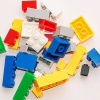### Colour Building

##### Age 11 to 14 Challenge Level:

Using only the red and white rods, how many different ways are there to make up the other rods?### Score

##### Age 11 to 14 Challenge Level:

There are exactly 3 ways to add 4 odd numbers to get 10. Find all the ways of adding 8 odd numbers to get 20. To be sure of getting all the solutions you will need to be systematic. What about. . . .### Tower of Hanoi

##### Age 11 to 14 Challenge Level:

The Tower of Hanoi is an ancient mathematical challenge. Working on the building blocks may help you to explain the patterns you notice.### How Old Am I?

##### Age 14 to 16 Challenge Level:

In 15 years' time my age will be the square of my age 15 years ago. Can you work out my age, and when I had other special birthdays?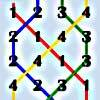### Oranges and Lemons, Say the Bells of St Clement's

##### Age 11 to 14 Challenge Level:

Bellringers have a special way to write down the patterns they ring. Learn about these patterns and draw some of your own.### Pinned Squares

##### Age 14 to 16 Challenge Level:

What is the total number of squares that can be made on a 5 by 5 geoboard?### Squares, Squares and More Squares

##### Age 11 to 14 Challenge Level:

Can you dissect a square into: 4, 7, 10, 13... other squares? 6, 9, 12, 15... other squares? 8, 11, 14... other squares?### Hidden Rectangles

##### Age 11 to 14 Challenge Level:

Rectangles are considered different if they vary in size or have different locations. How many different rectangles can be drawn on a chessboard?### Pattern Power

##### Age 5 to 14

Mathematics is the study of patterns. Studying pattern is an opportunity to observe, hypothesise, experiment, discover and create.### Magic Squares

##### Age 14 to 18

An account of some magic squares and their properties and and how to construct them for yourself.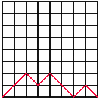##### Age 14 to 16 Challenge Level:

A walk is made up of diagonal steps from left to right, starting at the origin and ending on the x-axis. How many paths are there for 4 steps, for 6 steps, for 8 steps?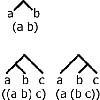### Counting Binary Ops

##### Age 14 to 16 Challenge Level:

How many ways can the terms in an ordered list be combined by repeating a single binary operation. Show that for 4 terms there are 5 cases and find the number of cases for 5 terms and 6 terms.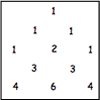### Investigating Pascal's Triangle

##### Age 7 to 14 Challenge Level:

In this investigation, we look at Pascal's Triangle in a slightly different way - rotated and with the top line of ones taken off.### Magic Squares II

##### Age 14 to 18

An article which gives an account of some properties of magic squares.### Triangles Within Pentagons

##### Age 14 to 16 Challenge Level:

Show that all pentagonal numbers are one third of a triangular number.### Top-heavy Pyramids

##### Age 11 to 14 Challenge Level:

Use the numbers in the box below to make the base of a top-heavy pyramid whose top number is 200.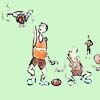### One Basket or Group Photo

##### Age 7 to 18 Challenge Level:

Libby Jared helped to set up NRICH and this is one of her favourite problems. It's a problem suitable for a wide age range and best tackled practically.